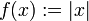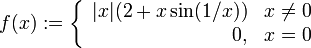# First derivative test and one-sided derivative test are incomparable

(diff) ← Older revision | Latest revision (diff) | Newer revision → (diff)$f(x) := x^2$,$c = 0$ local minimum Yes No, because both one-sided derivatives are zero$f(x) := |x|$,$c = 0$ local minimum Yes Yes$f(x) := \left\lbrace \begin{array}{rl} |x|(2 + x \sin(1/x)) & x\ne 0 \\ 0, & x = 0 \\\end{array}\right.$,$c = 0$ local minimum No Yes$f(x) := \left\lbrace \begin{array}{rl} |x|(2 + \sin(1/x)) & x\ne 0 \\ 0, & x = 0 \\\end{array}\right.$,$c = 0$ local minimum No No